Maths-
General
Easy

Question

# The sum of the radius and height of a cylinder is 21 cm and the T.S.A of the cylinder is 660 sq. cm. Find the height and volume of the cylinder ?Hint:

## The correct answer is: The height of the cylinder is 16 cm and the volume is 400π cm3.

### Explanations:Step 1 of 2:Let the height of the cylinder be h .Sum of the radius and height is 21 cm, so the radius will be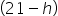cm.Given, TSA of the cylinder = 660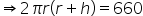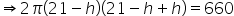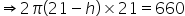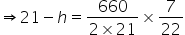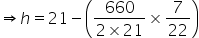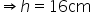So, r = 21 – 16= 5 cmStep 2 of 2:Volume of the cylinder =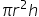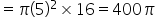cm3Final Answer:The height of the cylinder is 16 cm and the volume is 400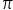cm3.#### With Turito Foundation.#### Get an Expert Advice From Turito.